# Appendix C:

## C1.0 Introduction

As discussed in Section 3.4, the Crank-Nicolson method is used to solve the dynamic solute transport equations (Equations (2) and (6)). The Crank-Nicolson solution of the main channel equation (Equation (6)) is the subject of this appendix. A similar discussion for the storage zone (Equations (2) is given in the main body of this report (Section 3.4.4).

The governing equation for the main channel is given by: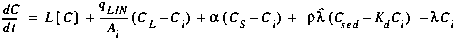(C1)

where L[C] is the finite difference approximation of the advection and dispersion terms, as given in Appendix B. In order to attain second order accuracy in both time and space, Equation (C1) is evaluated at the intermediate time level, j+1/2. To this end, the time derivative is approximated at the intermediate time level using a centered finite difference: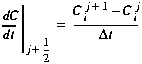(C2)

where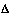t the integration time step [T] j denotes the value of a parameter or variable at the current time j+1 denotes the value of a parameter or variable at the advanced time
Furthermore, the right-hand side at j+1/2 is simply the average of the terms evaluated at times j and j+1. Combining this average with Equation (C2), Equation (C1) becomes: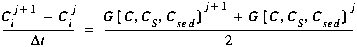(C3)

where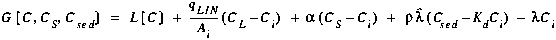(C4)

Because Equation (C3) is dependent on the solute concentrations in the neighboring segments at the advanced time level (Ci-1, Ci+1 at time j+1), it is not possible to explicitly solve for Cij+1. In the sections that follow, Equation (C3) is rearranged so that all of the known quantities (i.e. those at time j) appear on the right-hand side and all of the unknown quantities (i.e. those at time j+1) appear on the left. This rearrangement yields: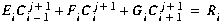(C5)

where E, F and G are matrix coefficients and R is a forcing function.

We now proceed to develop the matrix coefficients and the forcing function by examining each term in Equation (C3). In general, unknown quantities in each term contribute to the matrix coefficients while known quantities make up the forcing function. After going through each term, individual contributions are summed (see Section C4.0) to obtain E, F, G and R.

## C2.0 Left-Hand Side Of Equation C3 - Time Derivative

As noted above, Equation (C3) must be rearranged to develop the coefficients in Equation (C5). We begin this process by considering the left side of Equation (C3), the time derivative. Leaving the unknown concentration, Cij+1, on the left side and moving the known concentration to the right side, the contributions are given by: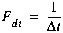(C6)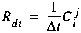(C7)

## C3.0 Right-Hand Side of Equation C3

The right side of Equation (C3) must also be rearranged such that the unknown quantities appear on the left side of Equation (C5) and the known quantities appear on the right. As shown in Equation (C4), G[C, CS, Csed] is made up of several terms. Each term in G[ ] is examined below.

In Equation (C4), the operator L[C] represents the finite difference approximation of the spatial derivatives describing advection and dispersion. The contribution of the advection term is the subject of this section, while the dispersion term is discussed subsequently.

Using the finite difference equations for the advection term (Equations (B7), (B11), and (B17)), we develop the contributions to the matrix coefficients shown below. Note that due to the presence of boundary conditions, the contributions depend on the type of segment for which Equation (C5) is developed.

### Interior Segments

Consulting Equation (B7), we see that: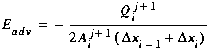(C8)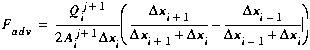(C9)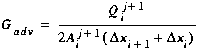(C10)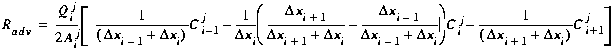(C11)

### First Segment

Using Equation (B11):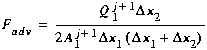(C12)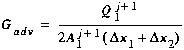(C13)(C14)

### Last Segment

Evaluating Equation (B17) at j+1/2 yields:(C15)(C16)(C17)

## C3.2 Dispersion

As with the advection term, the finite difference equations given in Appendix B (Equations (B9), (B14) and (B18)) are used to determine the contributions to the matrix coefficients.

### Interior Segments

Equation (B9), evaluated at j+1/2, yields: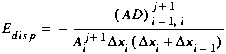(C18)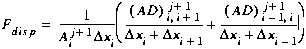(C19)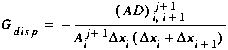(C20)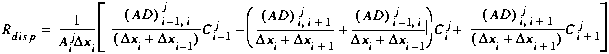(C21)

### First Segment

Using the finite difference equation for the first segment (Equation (B14)):(C22)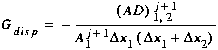(C23)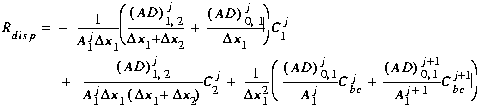(C24)

### Last Segment

Finally, Equation (B18) at j+1/2 yields: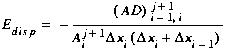(C25)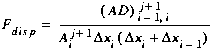(C26)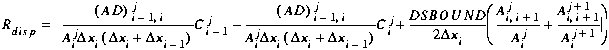(C27)

## C3.3 Lateral Inflow

The third term in G[ ], lateral inflow, is also evaluated at j+1/2: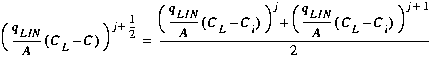(C28)

The contributions are thus: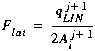(C29)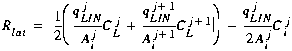(C30)

## C3.4 Transient Storage

The transient storage term is now evaluated at j+1/2: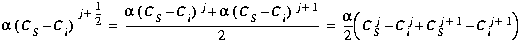(C31)

To decouple the governing transport equations (see Section 3.4.5), Equation (19) is used to eliminate CSj+1. Equation (C31) thus becomes: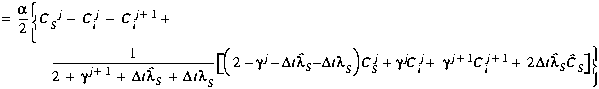(C32)

The contributions to the matrix coefficients are given by: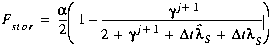(C33)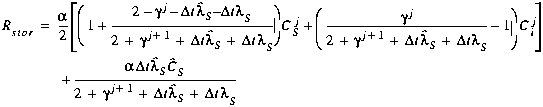(C34)

## C3.5 Sorption

The sorption term is evaluated at j+1/2: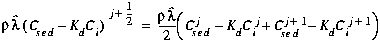(C35)

To decouple the governing transport equations (see Section 2.4.5), the Crank-Nicolson solution of the streambed sediment equation is used to eliminate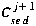. Equation (C35) thus becomes: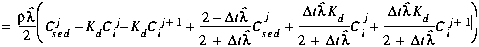(C36)

The contributions to the matrix coefficients are given by: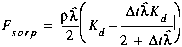(C37)(C38)

## C3.6 First-Order Decay

The final term in G[ ] represents first-order decay or production. This term is evaluated at j+1/2: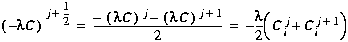(C39)

The contributions are given by: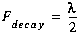(C40)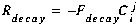(C41)

## C4.0 Matrix Coefficients

The matrix coefficients (E, F, G) and the forcing function (R) from Equation (C5) may now be developed by summing the contributions given in Sections C2.0 and C3.0, and multiplying byt:(C42)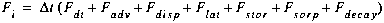(C43)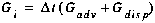(C44)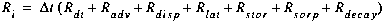(C45)